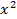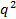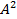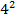# Functions and Graphing, Revision Notes# Functions and Graphing

A function relates an input to an output

If f(x) = - x + 1 then (x) = input - x + 1 = outputHere x just signifies the input it can be any letter or number

f(x) = - x + 1f(q) = - q + 1f(A) = - A + 1If our input x = 4 then we have

f(4) = - 4 + 1 = 13A function can also be expressed using y or f:x->

f(x) = - x + 1y = - x + 1f:x-> - x + 1The 3 expressions above mean the exact same thing

There are 3 types of basic graphs

Linear - This graph is a straight line

Expon...